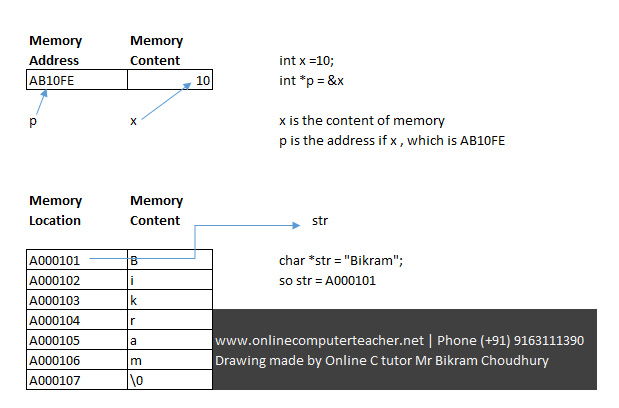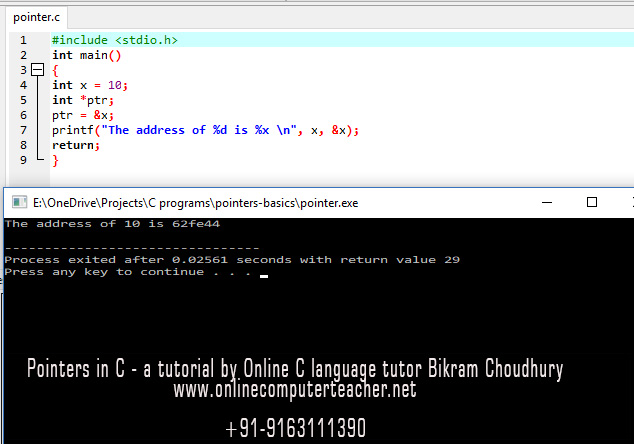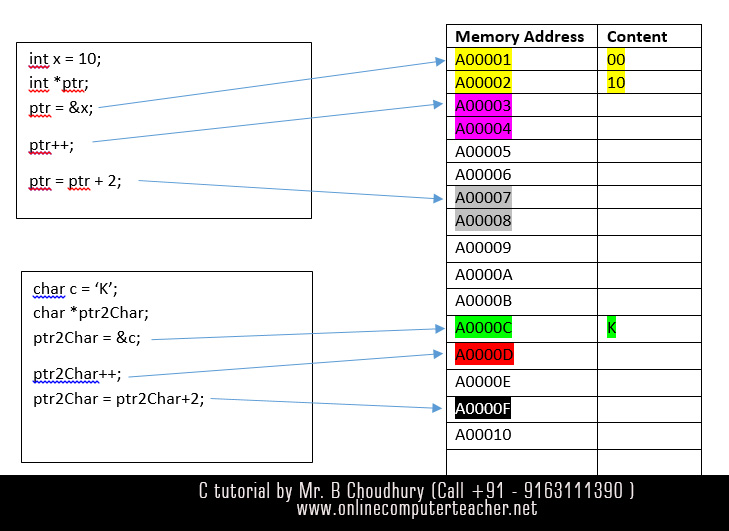# Pointers in C programming language – A tutorial for beginners

Learn the most difficult concept of pointers in C language, Pointers in C called nightmare for C language students or CSE (Computer Science & Engineering) students. All those who are new to programming & learning programming with C, stumble upon pointer concept.

Free C language tutorials >> Pointers in C >> C language Online Tutor >> Java Courses in Kolkata

### Concept of variable, pointers & memory address

A variable is stored in a memory location. The memory refers to the RAM / Random Access Memory of your computer. This topic is related to the study of computer science. So when we call 4GB/16GB RAM it refers to the Random Access Memory of your laptop or computer, in which a program is stored temporarily before execution. I am telling again this is purely a concept of computer science.

But you need to understand how program runs when you are learning C  programming concept.

When you are assigning a value 10 to a variable x or declaring a variable x ( e.g int x=10), then the value is stored in a memory location which is also called a memory address.

Address or memory address is usually denoted by hexadecimal characters 0 to 9 and A B C D E F as denoted in the picture, so A001BE can be a memory address.This image describes pointer concept in C programming language

### & ( address of ) operator and * (value at address) operator

So when we tell about a variable x as shown in the above picture, it actually points to a particular memory location. There are two parts attached to a memory location, a memory address and the content of that memory address. When we are telling about a variable x having a value 10 that means the content 10 stored at the memory address of variable x. So each variable x has two parts – address of x and content of x. Normally we deal with the content of a variable x. But when you’re dealing with pointers, then you are dealing with the address of a variable x, address of x is denoted by &x.

A pointer variable holds an address of any variable of a particular type. So if y is a pointer variable , which points to an integer is declared as
int *y or int* y both permissible.

So value at the address location denoted by y is denoted by * operator as *y, here y should be a pointer variable.

```int x = 10, *y;
y = &x;
in the above code y is a pointer variable
which points to an integer variable. Here
y points to address of x```

*y is the integer value stored at the memory address pointed by y. So if you print *y , you will see 10 printed.

```int x=10, *y;
y = &x;
printf("%d",*y); /* 10 will be printed */```

So *(&x) is x, it can be defined as “value at the address of x”

But pointer to an integer variable is different from a pointer variable to a float or pointer to character variable.

```int x=99, *y;
char *z, m='a';
float f=4.555,*ff;
y=&x;
z=&m;
ff=&f;```

char* is a pointer to a character or an address of a character in C. A character is usually takes 1 byte in memory. So my name in Bikram and it takes 6 characters and each character is stored in  a memory location.

Similarly, we can define int*, double* to indicate the address of an integer variable, address of a double type variable in C. A pointer is an address in the memory.

& is called the address of operator. A pointer should point to an address of a variable or type integer float double or char. Pointer to a string is basically a pointer to a character. If you need a teacher to learn C pointers, visit this link on C programming language courses

If a variable is x, address of x is defined as &x, & is a unary operator. The following simple program shows you how to find the address of an allocated variable x.

### A simple C program showing variable and memory address

```#include ;
int main()
{
int x = 10;
int *ptr;
ptr = &x;
printf(“The address of %d is %x \n”, x, &x);
return;
}
```

The output of the above program given below-run in DevC++.  %x is used to print hexadecimal addresses. %d is used to print /display in screen decimal integers in CPointer concept in C, a tutorial by Bikram Choudhury, www.onlinecomputerteacher.net

### Pointer Arithmetic – add integer to pointers

An integer value can be added to a pointer variable or can be subtracted from a pointer, but when we do the addition or subtraction this way actually what happens shown below.increase pointer variable in C, a tutorial by Online C language tutor Bikram Choudhury

Say the pointer variable is of type int & pointing to an integer variable. An integer variable takes 2 bytes /4 bytes in memory. So every integer is stored in memory takes 2 bytes, though actually how many bytes an integer will take,  depends on compilers. Anyway so when I am adding 1 to a pointer variable of type int* by ptr++, then the pointer should point to next integer, so two bytes to be added to the pointer variable ptr.

But when the pointer variable is of char*, i.e. pointer to a character variable, & then if we increase the pointer by 1,then the pointer variable ptr2Char points to the next Byte or next memory address, because a character takes 1 byte to be stored in memory.

Every memory location is capable of storing one character because every memory location can store 1 byte of data in it, as shown in the figure above. So when you add 2 to the pointer variable of ptr2Char of type (char *) / character then 2 bytes to be added. So number of bytes skipped depends on the data type of the pointer, like integer float or character or double.

Even if you add only one to a pointer of type float, it will skip 4 bytes in memory.

```float varf = 9.8;
float *ptrF = &varf;
ptrF++; // 4 bytes will be skipped in Memory```

### Void Pointer

The void pointer/ void* in ANSI C and C++ denotes a generic pointer type. A pointer to void can store the address of any variable of type int, char, double etc.But it cannot point to a function and cannot perform the role of function pointer. In ANSI C, void pointer of type void* is implicitly converted to any other pointer type (int *, char* .. ) on assignment, but it must be explicitly cast if de-referenced.

```int i = 3;
void* vp1 = &x;
int* p2 = vp1;   // void* automatically or implicitly converted to int* in ANSI C (but not in C++)
```

So now p2 is an integer pointer, *p2 is an integer if you print it using pritf();
But if you want to de-reference void pointer vp1 then you should first convert it to integer pointer by (int*)

`int d = *(int*)vp1;  // when de-referencing, it is not done automatically implicitly`

• C++ does not allow this implicit pointer conversion (e.g void* to char*)
• C++ does not allow the implicit conversion of void* to other pointer types like int* or char*.

### Dangling pointer / Uninitialized pointer

A dangling pointer is a pointer that does not point to a valid variable / memory location, and consequently may make a program crash or behave oddly. In C programming languages, pointers that are not specifically initialized may point to unpredictable addresses in memory.
The following example code shows a dangling pointer:

```int *ptr = malloc(sizeof(int)); /* (undefined) value of some place on the heap */
char *p;       /* dangling (uninitialized) pointer */
*ptr = 10;     /* This assignment will be successful if malloc() does not return NULL. */
*p = 'Y';   /* undefined behavior, not allowed. Program can crash */
```

The character pointer p may point to anywhere in the memory, *p = ‘Y’ can trigger segmentation fault behave in undefined way, or corrupt a memory location.

### Some pointer assignment which are not permitted

A constant string can be initialized as char* str = “Bikram\0”; but no memory is allocated for str. If we try to write to str again, then it may cause a segmentation fault since memory has not been allocated explicitly.

```char* str = “Bikram”;
char* str1 = “Bikram\0”;
fscanf(stdin,”%s”,str);
```

the last statement would cause a problem, this type of re-assignment of strings are not permitted in C

```char *ptr = “Hello World”; /* permitted */
*ptr = “Hello”; /* You cannot re-assign a string */
```

For more information visit the link on string handling in C using pointers

### malloc( ) – allocate memory dynamically

So you should allocate memory first and then copy the string to the location. To allocate a block of memory to hold a string, we use the malloc function from <stdlib.h>.

The malloc(int n) returns a pointer to a block of n bytes. A string with n characters requires n+1 bytes (n for the n charactersa and extra 1 byte to store the NULL character at the end which is ‘\0’ character). So the string “Bikram” will take 6 characters + ‘\0’, i.e 7 characters. So you need to allocate 7 characters using malloc() function.

```char *str = malloc( 5 * sizeof(char) );
strcpy(str,"Bikram");
```

malloc allocates memory and holds it the memory location even after running it, called the “dynamic heap” and unless memory is explicitly freed using free() function call, the use of malloc() stays even after leaving the scope of the code.

Using the character notation we can do the same job this way :

```char str;
strcpy(str,”Bikram”);
```

In this case, 7 bytes is allocated from the run time stack and str is no longer available once it is out of scope.